# Network Flows and their applicability

## Introduction

In this article, we will take a look into some sets of algorithms that are usually referred to as “the hammer to solve all problems”: Network Flow. Although that might not be true, they can be used to solve plenty of hard problems. In this article, my objective is to present network flows and give conceptual solutions to the problems described.

Network Flow is part of the graph theory toolbox and it is used to model problems such as transportation networks, scheduling/planning, and matching of resources to name a few. With today’s libraries, we can use a simple method call to get the answer so that shifts my focus towards modeling the problem. Modeling in this context can be the hardest part of the whole solution as Network Flows are a general tool and can be used to model a whole spectrum of problems.

Before we begin let me define some concepts. Think about your apartment piping system. Each pipe has a maximum capacity of how much water can go through it. It wouldn’t be wise from the building design to try and push more water through your apartment pipes, as it would damage them and it would cause leakage. If we want to abstract this apartment building we would represent an object that uses water (tap, dishwasher, showerheads, etc.) as a node in a graph. The pipes are the edges of our graph and they have a defined maximum capacity exactly as the pipes. A network flow is a flow from the water motor in the basement of the building to all the apartment appliances, subject to respecting all the constraints of each pipe.

There exist a number of flavors of network flows that differ by their objective. One of the most used ones is maximum flow. This algorithm finds the maximum feasible flow through a network respecting the capacities of each edge. This can be useful in a number of scenarios as the water system in our homes, traffic in a city, and as we will see, to seemingly unrelated problems.

## Simple example

Let us start with our first application. Given a graph G=(V, E) we are required to find how many unique paths exist from node to node j (i ≠j). A unique path (i.e. edge-disjoint path) is a path does not share an edge with another path. The only extra step needed to solve this problem is to set the capacity of each edge to one and run one of the maximum flow algorithms from your favorite library (e.g. python networkx). Why does this work? The core of this solution is where we put the capacity to 1 for each edge on the graph. The interpretation is that if we have ants starting at node i, we will only allow 1 ant to pass through an edge. The maximum flow value gives you that exact number of how many ants did arrive at node j, respecting our constraint of one ant per edge.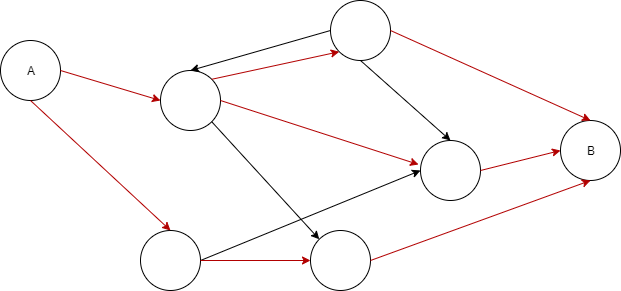edge-disjoint paths (red) from A to B in a given directed graph G=(V, E)

A similar but trickier example would be if we change the constraint of the problem to be unique nodes rather than unique paths. This means that multiple ants can reuse an edge multiple times but only one ant can pass through one node. The trick here is to transform the graph (or the problem) to an edge-disjoint problem as we did before. The way we can do this is by first duplicating every node except and j; let’s call them and k`. We add edges (k, k`) with capacity oneand every other edge we set it to infinite capacity.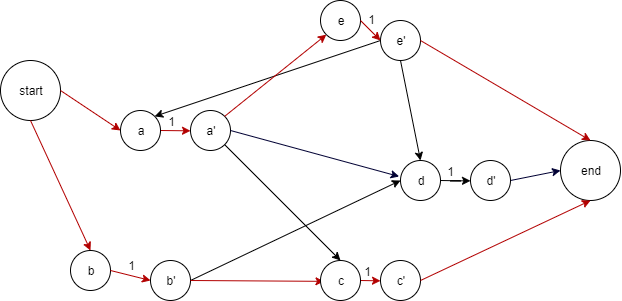node-disjoint paths (red) from start to end in a given directed graph G=(V, E). Note that the graph is the same as before, with the addition of the duplicate nodes. We can only reach the end with 2 different paths without going in the same node twice. This is not a unique solution, however, there are no more than 2 paths.

## Assigning employees to projects

Now that we gave two simple examples of network flows let us solve a more interesting problem, namely a matching problem. A software engineering company has 5 employees and they have 5 projects. They want to assign 1 project to each of their employees given that a project has a set of programming languages required (i.e. Java, Python, R, etc.) and each employee knows multiple languages. This problem is known as the bipartite matching problem. A bipartite graph is a graph G=(N₁ ∪ N₂, E) such that every edge connects a node in N₁ with N₂. N₁ in our problem are the workers, and N₂ is the job we are trying to match workers to.

We begin our solution by first adding two special nodes, called source (s)and sink (t). We connect the source(s) every node (employee) in N₁ and every node (project) of N₂ to sink(t). The edges between the sets of employees N₁ and the set of projects N₂ should be as such that (e, p) ∀ w ∈ N₁, p ∈ N₂ only exists when an employee has the language knowledge given in the project requirement. Additionally, every edge in this graph should have a capacity of one since we are trying to uniquely match workers to projects. Running the maxflow algorithm from source (s) to sink (t) will give us the matchings between employees and projects.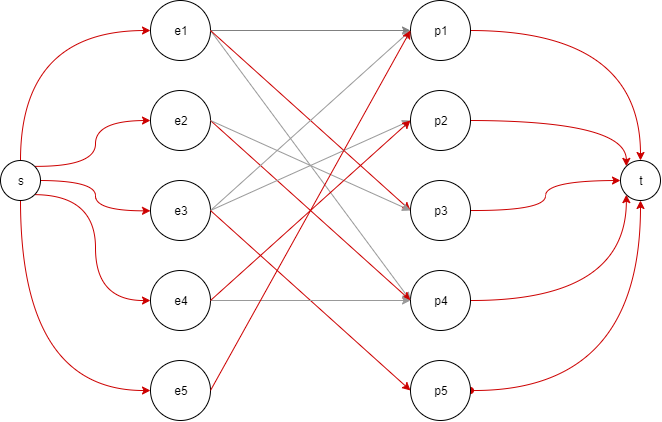A bipartite graph for the matching of 5 employees with the 5 projects. The red edges are the matchings.

Note: In our example with 5 workers and 5 projects we could easily do it by hand. However, let’s say that we build an automated system wherein the beginning of each month we do this matching and we have substantially more employees and projects. As we will see later things are not that easy as described in this example in practice.

## Extending our problem

Now let us extend the example with some more constraints as things are not that easy in real life. Same as before we have n employees and m projects. For each employee, we have a set of programming languages he knows and a seniority rank x (a number between [1, 5] where 1 is junior and 5 is expert) of the expert knowledge. Each project, on the other hand, has a requirement document that lists the languages that are needed to work on the project, along with a rank y ([1, 5] where 1 is simple and 5 experts only) which is the “difficulty” of the project. The difficulty of a project means that an employee of seniority rank x can only work on a project of the same rank y or at most 2 ranks below rank y (i.e. [x-2, x]). Additionally, each project has a budget that shouldn’t be exceeded by the wages of the employees working in the project. (table)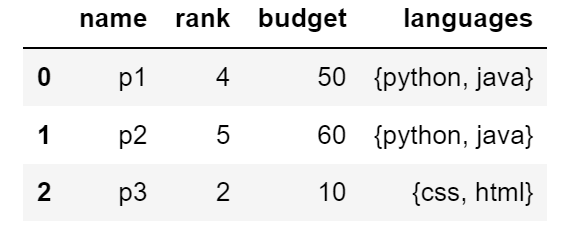Information for employees and projects. As we can see we cannot have a perfect matching (since |emp| > |proj|). Additionally, some projects have a budget less than an employee salary (namely p3).

There are multiple ways to model this kind of problem using network flow. However, we are interested in complying primarily with the budgetary constraints of the projects. That means a project can have multiple employees, however, the budget of the project needs to cover for all employees assigned fully. We start modeling by giving each employee a node w and each project a node p. We add edges (w, p) if and only if rank x – rank y ≤ 2, and the requirements of the project match the knowledge of the workers.

Matching mechanism: we define matching as the jaccard_index(req_proj, know_worker) ≥ 50%, but this may vary depending on requirements.

Each of these edges should have the capacity equal to the wage of the worker. Lastly, we connect the source(s) to each worker with an infinite capacity (or (x * wage), depending on how many projects can an employee work simultaneously). This can be interpreted as a billing system because a worker can work on multiple projects at once. Each project will be connected to the sink(t) with a capacity equal to the budget of that project.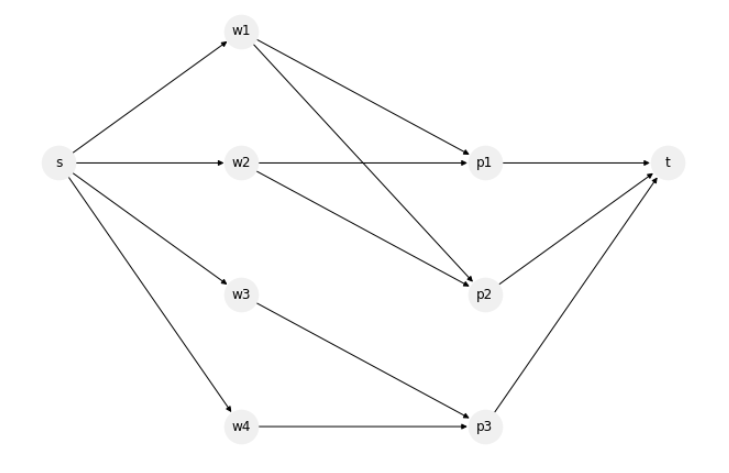The bipartite graph of employees and projects, along with 2 special nodes (s) and (t).

Now we can run one of the network flow algorithms from source(s) to sink(t) and to get the optimal matching given the constraints and definition. This may sometimes need some post-tweaking depending on your definitions and constraints, however, this is a simple way to model this problem.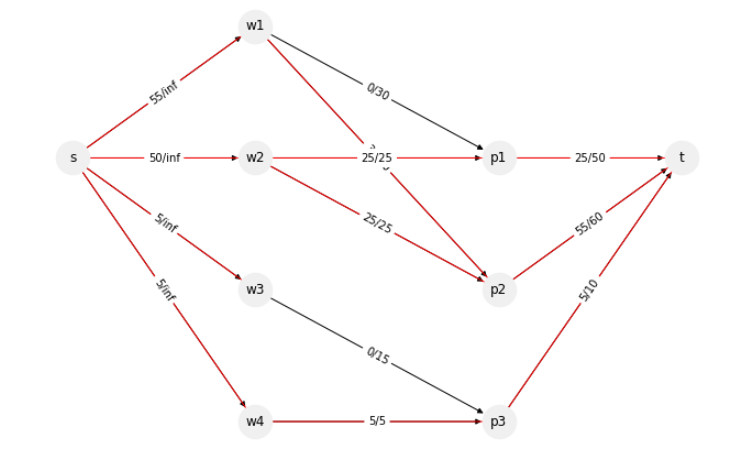The solution to the extended problem. In this instance, the algorithm I ran was max_flow_min_cost (provided by networkx library). The only difference was that the weights between (w, p) are equal to the wage of the worker. After running the algorithm we may need to filter a not saturated (w, p)-edge. Per definition, we only accept saturated (w, p)-edges (i.e. the budget of the project needs to cover for all employees assigned fully).

## Conclusion

Network flows are a great area of research for solving a huge variety of problems. This article was intended to give anintroductionto modeling problems in this context. As noted in the introduction, there exist a great number of optimized algorithms for solving maxflow (or any other flavors), and progress is still being made. However, if your focus is more towards the applicability, you would find that modeling a problem in network flow terms is tricky, depending on constraints and abstraction of the problem.

Wrapping my first post. If you enjoyed this introduction to network flow be sure to follow as I intend to write more examples in the future. Feel free to leave any feedback. Find me on LinkedIn.

-Armando

This blog was originally published on https://blog.arinti.be/a-summary-of-knowledge-based-job-ecommender-systems-2aa2fa46e56a

Blog